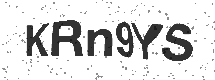## Audio Quiz: Type The Telephone Number

05:00

1. What is this number?

2. What is this number?

3. What is this number?

4. What is this number?

5. What is this number?

6. What is this number?

7. What is this number?

8. What is this number?

9. What is this number?

10. What is this number?